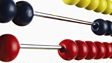Two students take part in the Maths Channel Quiz Show. They take it in turns to answer some everyday maths problems using addition and subtractions.This clip is from:
The Maths Channel, Year 2: Subtractions
First broadcast:
11 September 2007

Children could roll a die twice to get two numbers that they would then add together and create an addition calculation. They could then use the numbers to write a subtraction. Alternatively, children could be given three numbers on cards and be asked to arrange them into calculations for addition and then subtraction.